# Speed facts for kids

Kids Encyclopedia Facts
Speed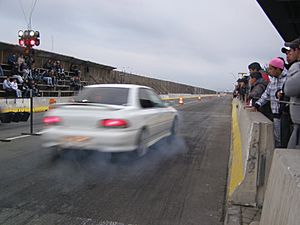Speed can be thought of as the rate at which an object covers distance. A fast-moving object has a high speed and covers a relatively large distance in a given amount of time, while a slow-moving object covers a relatively small amount of distance in the same amount of time.
Common symbols
v
SI unit m/s, m s−1
Dimension L T−1

Speed is the distance that an object moves in a given amount of time. Speed is a measure of how fast something is moving. The average speed of an object in a certain time is the distance the object traveled divided by the time.

## Finding speed

To find speed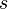$s$,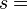$s =$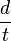$d \over t$

where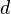$d$ is the distance and$t$ is the time that has gone by.

## Units of measurement for speed

There are many units of measurement for speed. For example, an object's speed can be measured in

## Changing speed

When an object changes speed, it gets faster or slower. If the speed of the object increases, it is called acceleration. If the object gets slower, and the speed decreases, it is called deceleration, or negative acceleration.

## Related pagesSpeed Facts for Kids. Kiddle Encyclopedia.